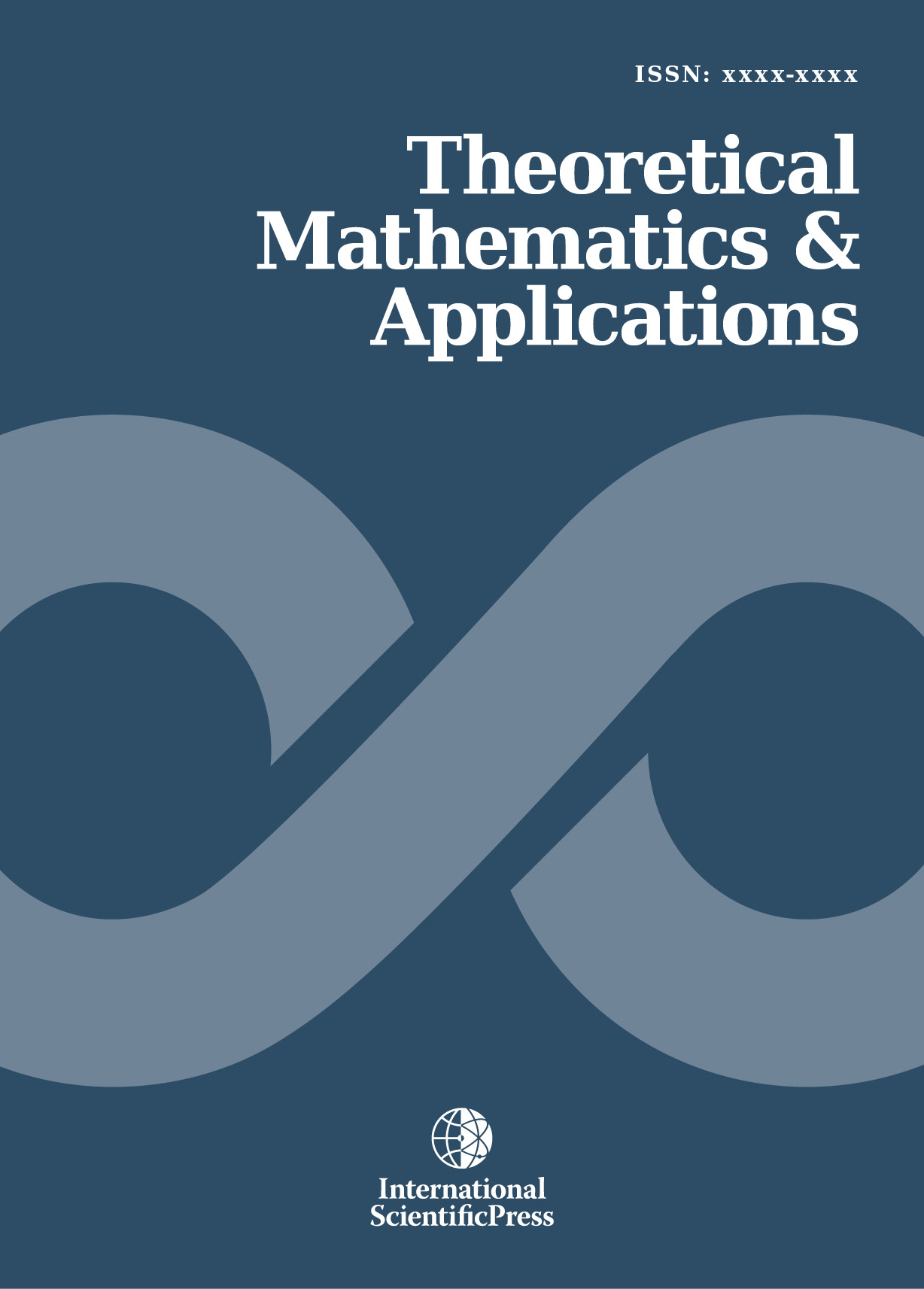# Theoretical Mathematics & Applications

#### On computing Gaussian curvature of some well known distribution

•[ Download ]
• Times downloaded: 1019
• Abstract

The objective of this paper is to provide a deeper and broader understanding of the meaning of Gaussian curvature. It also suggests some more general alternative computational methods than Kass, R.E. and Vos, P.W. [1, 2]. It is not intended to compare the superiority of the four formulas to be used in computing the Gaussian curvature. We define the coefficients of the expected Fisher Information Matrix as the coefficients of the first fundamental form see Lehmann, E.L. . Four different formulas adopted from Struik, D.J.  are used, and labeled here as (A), (B), (C), and (D). It has been found that all four of these formulas can compute the Gaussian curvature effectively and successfully. This is demonstrated by four commonly used examples. Also it would be interested to compare our methods with Kass, R.E. and Vos, P.W. approach [1, 2].ISSN: 1792-9687 (Print)
1792-9709 (Online)